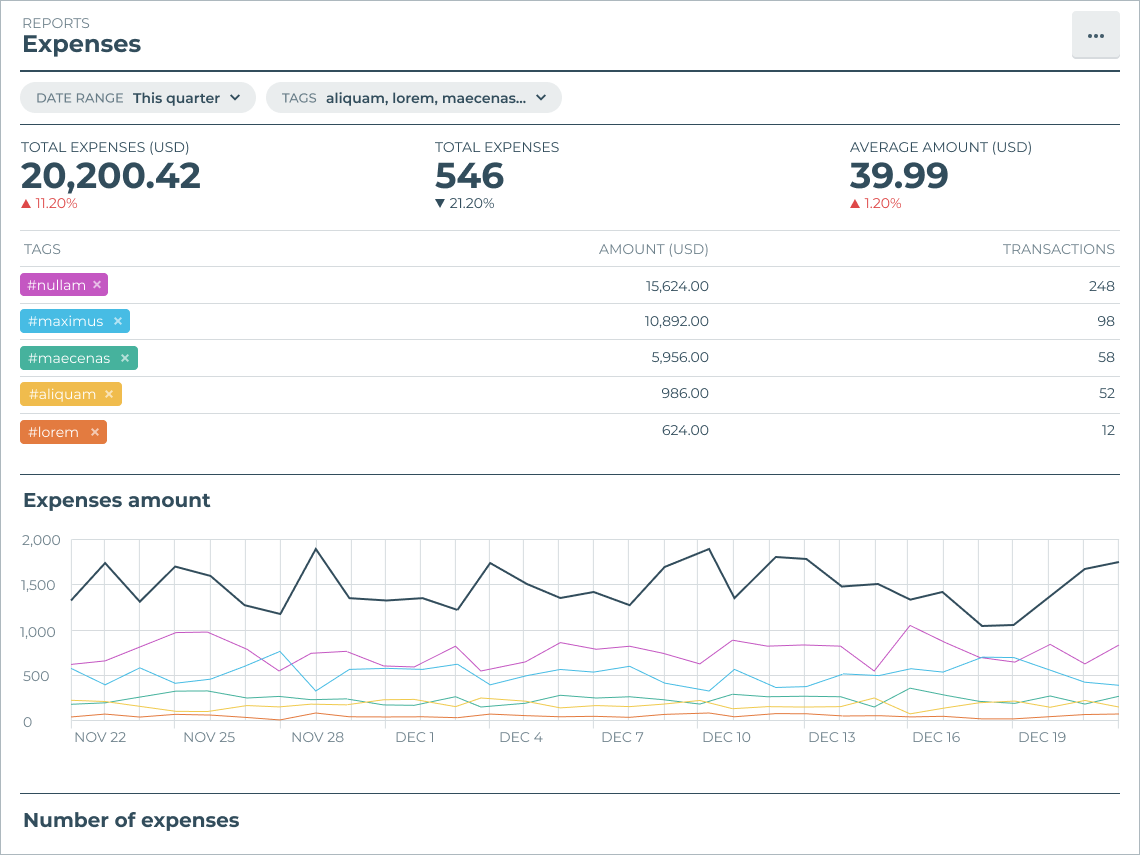# Expenses report

The expenses report gives you an overview of your expenses. All amounts include tax (gross amounts).The expenses report displays:

• Total expenses (currency) – Your total expenses. The total amount registered in your Quaderno account as expenses for the given period.
• Total expenses – The total number of expenses registered in your Quaderno account for the given period.
• Average amount – The average amount of an expense.
• Expenses amount – The total sum of expenses on a given day.
• Number of expenses – The number of expenses on a given day.